Select Page

# CBSE Maths 12 Science MCQ Application of Derivatives Answers in English

CBSE Maths 12 Science MCQ Application of Derivatives Answers in English to enable students to get Answers in a narrative video format for the specific question.

Expert Teacher provides CBSE Maths 12 Science MCQ Application of Derivatives Answers through Video Answers in English language. This video solution will be useful for students to understand how to write an answer in exam in order to score more marks. This teacher uses a narrative style for a question from Application of Derivatives not only to explain the proper method of answering question, but deriving right answer too.

Please find the question below and view the Answer in a narrative video format.

Question:

## Similar Questions from CBSE, 12th Science, Maths, Application of Derivatives

Question 1 : Find approximate value of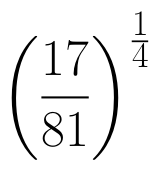(View Answer Video)

Question 2 : The slope of the normal to the curve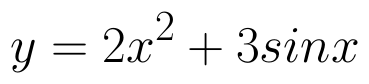at x = 0 is :

Question 3 : For the curve, y=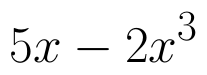if x increases at the rate of 2 units/sec, then how fast is the slope of curve changing when x=3? (View Answer Video)

Question 4 : Find the approximate change in volume V of a cube of side x meters caused by increasing the side by 1%. (View Answer Video)

Question 5 : If the radius of a sphere is measured as 9 m with an error of 0.03 m, then find the approximate error in calculating its surface area. (View Answer Video)

### Vector Algebra

Question 1 :  Find the scalar quantities from the following:
(i) 10 kg          (ii) 2 m north-west          (iii)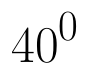(iv) 40 W         (v)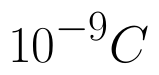(vi)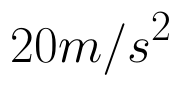(View Answer Video)

Question 2 : For given vectors,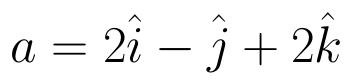and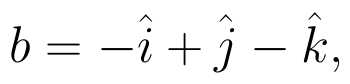find the unit vector in the direction of the vector a + b. (View Answer Video)

Question 3 :  Write the value of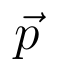for which the vectors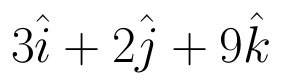and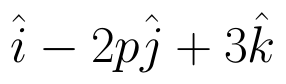are parallel vectors.  (View Answer Video)

Question 4 : Write the position vector of the point which divides the join of points with position vectors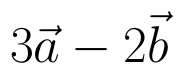and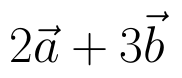in the ratio 2:1.  (View Answer Video)

Question 5 : Find the magnitude of two vectors a and b having the same magnitude and such that the angle between them is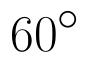and their scalar product is 1/2. (View Answer Video)

### Differential Equations

Question 1 : If x cos(a + y) = cos y, then prove that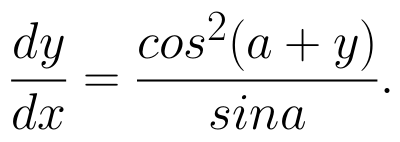Hence show that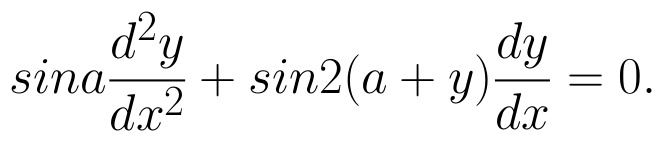(View Answer Video)

Question 2 : Write the differential equation representing the curve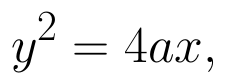where a is an arbitrary constant. (View Answer Video)

Question 3 : Write the differential equation formed from the equation y = mx + c, where m and c are arbitrary constants.

Question 4 : Solve the differential equation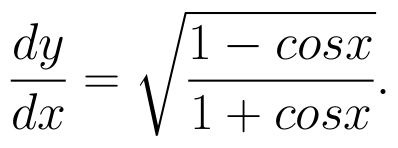(View Answer Video)

Question 5 : Find the solution of the differential equation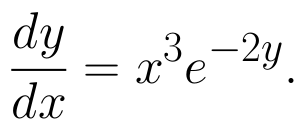(View Answer Video)

### Inverse Trigonometric Functions

Question 1 :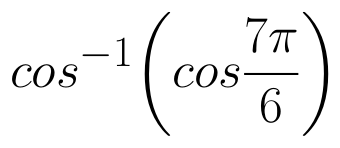is equal to :
Question 2 : Write the value of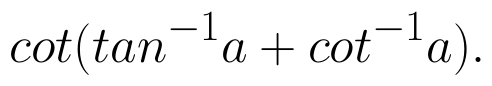(View Answer Video)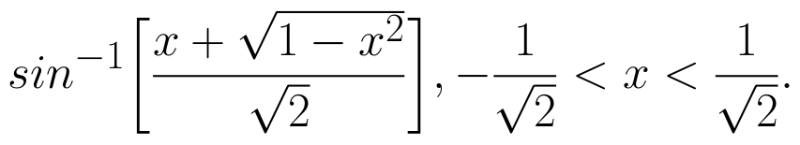(View Answer Video)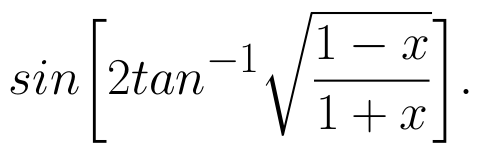(View Answer Video)
Question 5 : If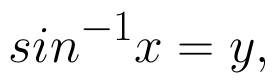then value of y lies between.....? (View Answer Video)